# ChIPS - drawing lines between sets of (x,y) pointsChIPS Threads (CIAO 4.11 ChIPS v1)

## Overview

#### Synopsis:

This thread is intended to give a simple demonstration of the basic interactive capabilities of ChIPS: plot data from a file, add labels, adjust the axes of a plot, change the attributes of an existing object such as a curve, the ability to undo previous changes, and hardcopy output.

Last Update: 15 Dec 2015 - Updated for CIAO 4.8.

## Introduction

The Starting ChIPS thread describes how to start ChIPS. Please see the ChIPS GUI section of that thread for information on the ChIPS GUI.

The data used in this thread is available in the chips_data.tar.gz file.

```unix% ls -1 chips/curve_line/
pos.dat
```

The plots used in this thread are based on the data in the text file pos.dat which gives the location of Chandra, in terms of latitude, longitude, and altitude, for a range of times. The contents of the file look like:

```unix% head pos.dat
# utime longitude latitude altitude
1201380180 162.9000000000 39.80000000000 95159.0
1201380420 162.0 39.60000000000 94901.40000000
1201380600 161.4000000000 39.50000000000 94707.30000000
1201380720 160.9000000000 39.40000000000 94577.60000000
1201380840 160.5000000000 39.40000000000 94447.60000000
1201381020 159.9000000000 39.20000000000 94251.90000000
1201381140 159.4000000000 39.20000000000 94121.10000000
1201381260 159.0 39.10000000000 93988.90000000
1201381380 158.6000000000 39.0 93858.50000000
unix% dmlist pos.dat cols

--------------------------------------------------------------------------------
Columns for Table Block pos.dat
--------------------------------------------------------------------------------

ColNo  Name                 Unit        Type             Range
1   utime                             Real8          -Inf:+Inf
2   longitude                         Real8          -Inf:+Inf
3   latitude                          Real8          -Inf:+Inf
4   altitude                          Real8          -Inf:+Inf
```

The longitude and latitude columns are in degrees, the altitude is in kilometers, and the utime column gives the number of seconds since 00:00:00 on January 1, 1970, Coordinated Universal Time (UTC). These values were obtained from a Chandra tracking page at NASA that is no longer available. The Chandra public outreach site has a page on tracking Chandra and other NASA satellites.

## A simple plot

The make_figure command can be used to create a quick plot of a file. Here we use it to plot up the latitude and utime columns of the file:

```chips> make_figure("pos.dat[cols utime, latitude]")
```

### Figure 1: Latitude versus time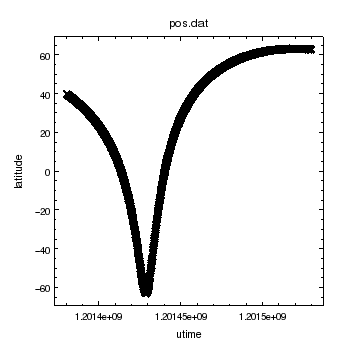### Figure 1: Latitude versus time

The make_figure command has created a plot of the latitude versus utime columns, and set up the labels (axes and titles) based on the column and file names. The data points are drawn as a curve, indicated by a symbol at each point and with the points connected by straight lines (although this is not obvious in this figure due to the close spacing of the points). Figure 2 shows the plot when the curve is drawn only with lines.

Properties of the plot - such as the symbol style to draw or line color to use - can be changed once the figure has been created (they can also be changed before creating the plot). Since there are many points close together we decide to remove the symbols from the curve using:

```chips> set_curve(["symbol.style", "none"])
```

### Figure 2: Latitude versus time (no symbols)### Figure 2: Latitude versus time (no symbols)

The latitude versus time plot (Figure 1) after turning off the symbol display for the curve.

Further changes can be made and - thanks to the support for undo and redo - be removed if neessary:

```chips> set_curve(["line.color", "yellow"])
chips> undo()
```

These commands change the line used to connect the latitude and time values to yellow and then removes this change - so that the display at this point matches Figure 2.

The following commands change the line color, axis labels, and the plot title. The print_window calls create postscript (plot1.ps), PNG (plot1.png), and PDF (plot1.pdf) versions of the display respectively. The "hardcopy" version of this plot is shown in Figure 3. The use of the save_state command is described below.

```chips> set_curve(["line.color", "red"])
chips> set_plot_title("Chandra's location")
chips> set_plot_xlabel("Time [s]")
chips> set_plot_ylabel(r"Latitude [\textdegree]")
chips> set_xaxis(["tickformat", "%z"])
chips> print_window("plot1")
chips> print_window("plot1.png")
chips> print_window("plot1.pdf")
chips> save_state("plot1.state")
```

### Figure 3: Latitude versus time (hardcopy version)### Figure 3: Latitude versus time (hardcopy version)

The default colors used for foreground and background elements depend on the output format: white on a black blackground when drawn on screen and black on a white background when a hardcopy verion is creates with print_window.

Objects who have their color set to a value other than default, such as the line in this figure, will be drawn in that color in both the screen and hardcopy versions.

By using the %z format for the tickformat of the X axis, the labels are drawn using exponential notation rather than as 1.2014e+09, as in Figure 2.

## How do I know what is what?

ChIPS provides a rich variety of objects (such as curves, histograms, labels) for creating plots, a large number of attributes (such as "line color" and "symbol style") for controlling the appearance of the plots, and a powerful system for determining the default objects at a given time (currency). However, you do not need to fully understand these ideas to use ChIPS to make plots!

The ChIPS GUI makes it easy to explore and modify your ChIPS visualizations.

### What parts of a curve I can change?

Figure 1 shows the default properties of a curve, which is that the points are connected by a solid line and marked by a symbol in the shape of a cross. To find out what attributes you can change, once you have created a curve, you can use the get_curve routine:

```chips> print(get_curve())
depth = 100
err.caplength = 10
err.color = default
err.down = False
err.left = False
err.right = False
err.style = line
err.thickness = 1.0
err.up = False
id = None
limit.length = 0.0500000007451
limit.override = True
line.color = red
line.style = 1
line.thickness = 1.0
stem = None
symbol.angle = 0.0
symbol.color = default
symbol.fill = True
symbol.size = 5
symbol.style = 0
```

The two lines in bold indicate the attributes of the curve we changed using the set_curve routine (the bold font has been added here for display purposes and does not appear in the actual screen output). Some attribute values can be set as either a string or numeric value; for such attributes the print out will use the numeric value, which is why the symbol.style attribute has a value of 0 and not none.

If you have started the GUI - either with the show_gui command or from the Show GUI entry in the right-mouse-button menu of the ChIPS window, then you can view and modify all the attributes of the objects. For the curve in this plot we would see Figure 4.

### Figure 4: The ChIPS GUI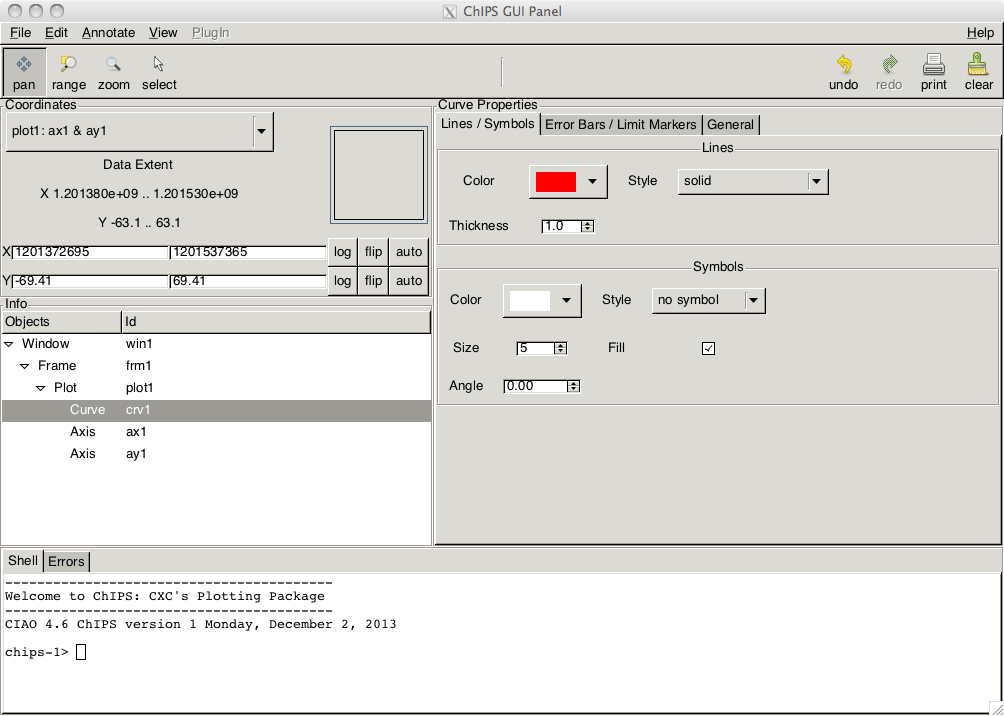### Figure 4: The ChIPS GUI

The object attributes can be changed in the GUI, with the changes being reflected immediately on screen. To change the object being modified, either select the name in the Info frame or double click on the object in the ChIPS window.

The return value from get_curve can be used in set_curve as an alternative way to change the attributes. For instance, the following code changes the curve in Figure 3 to be drawn in a thick blue line:

```chips> ci = get_curve()
chips> ci.line.thickness = 4
chips> ci.line.color = "blue"
chips> set_curve (ci)
```

### Figure 5: Using get_curve and set_curve### Figure 5: Using get_curve and set_curve

The set_curve call has changed the curve to be drawn with a blue color and with a thicker line.

Since both changes were made by a single call to set_curve, they will both be removed by a single call to undo:

```chips> undo()
```

The properties of other objects can be found and changed using the get/set routines; for instance get_xaxis, set_xaxis, get_yaxis, set_yaxis, get_plot, and set_plot. It is also possible to change the properties of objects before they are created (using the preference system) or as they are created (by adding the attribute values to the add_<object> calls). These will be described in later threads, and are also demonstrated below (in creating Figure 9 and Figure 6 respectively).

The get_preference routine can be used to find out what the default parameters for an object are: these are the values that will be used the next time an object is created. The return value is a string, and the output below shows the default values for a curve:

```chips> print(get_preference("curve"))
curve.stem             : crv
curve.depth            : default
curve.line.color       : default
curve.line.thickness   : 1
curve.line.style       : solid
curve.symbol.color     : default
curve.symbol.style     : cross
curve.symbol.size      : 5
curve.symbol.angle     : 0
curve.symbol.fill      : true
curve.err.color        : default
curve.err.thickness    : 1
curve.err.style        : line
curve.err.up           : on
curve.err.down         : on
curve.err.left         : on
curve.err.right        : on
curve.err.caplength    : 10
curve.limit.length     : 0.05
curve.limit.override   : true
```

### What objects have been created?

The info command returns a string that describes what objects have been created:

```chips> print(info())
Window [win1]
Frame [frm1]
Plot [plot1]   (0.15,0.15)  .. (0.90,0.90)
Border bottom [bx1]  top [bx2]  left [by1]  right [by2]
Curve [crv1]
X Axis [ax1]
Y Axis [ay1]
```

This is read as: there is a window called win1, a frame called frm1, a plot called plot1, four borders (called bx1, bx2, by1, by2), X and Y axes called ax1 and ay1 respectively, and a curve called crv1. These objects were all created automatically by the make_figure call. It is possible to create these individually (there are add_<object> calls), and to use different names (e.g. instead of crv1). in later threads.

The indentation in the output of info indicates the hierarchy of the objects; the curve "belongs to" the plot, which itself belongs to a frame. This hierarchy becomes important once you start adding multiple objects (for instance multiple plots), or when you want to delete an item (since everything that belongs to it will also be deleted). For instance, if we delete the plot then the curve will also be deleted but the frame and window will remain (the deletion can be undone to restore the plot):

```chips> delete_plot()
chips> print(info())
Window [win1]
Frame [frm1]

chips> undo()
chips> print(info())
Window [win1]
Frame [frm1]
Plot [plot1]   (0.15,0.15)  .. (0.90,0.90)
Border bottom [bx1]  top [bx2]  left [by1]  right [by2]
Curve [crv1]
X Axis [ax1]
Y Axis [ay1]
```

This hierarchy is also available in the ChIPS GUI, as shown in Figure 4.

In this section we will add more data - the longitude versus time arrays - to the plot and add some annotations, in the form of labels, to identify the curves.

```chips> add_curve("pos.dat[cols utime,longitude]", ["symbol.style", "none"])
```

### Figure 6: Adding a curve to a plot### Figure 6: Adding a curve to a plot

The add_curve call has been used to add a second curve to the plot. The data columns user were longitude versus time.

The default symbol style for curves has been over-ridden in the add_curve call, rather than by using a set_curve call, as was done with Figure 1. Also note that the y-axis scale has automatically increased to include the new data (the previous range was approximately -60 to 60 and it is now approximately -160 to 160).

The following commands change the y-axis label (since it is no longer correct) and adds labels to indicate which curve is which. Note that the first add_label call overrides the default color of the label in a similar manner to the add_curve call above:

```chips> set_plot_ylabel(r"Position [\textdegree]")
chips> add_label(1.20148e9, 100, "Latitude", ["color", "red"])
```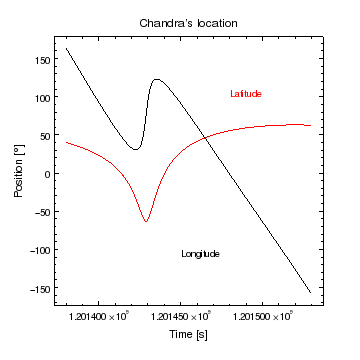Labels have been added to identify the curves.

The size and positions of the labels are not ideal, so we shall change them. First we use the get_label call to find out the current size; since we are only interested in a single value we just get that value, but we could have used print(get_label()) instead. The set_label call changes both the curves at once since it uses the special value "all" for the optional name argument.

```chips> get_label().size
12
chips> set_label("all", ["size", 18])
chips> print(info())
Window [win1]
Frame [frm1]
Plot [plot1]   (0.15,0.15)  .. (0.90,0.90)
Border bottom [bx1]  top [bx2]  left [by1]  right [by2]
Curve [crv1]
X Axis [ax1]
Y Axis [ay1]
Curve [crv2]
Label [lbl1]
Label [lbl2]
```

The output of info shows that the two labels are called lbl1 and lbl2. As the numbering is sequential lbl1 is the first label ("Latitude") and lbl2 the second one ("Longitude"). These names can be used to specify which label is to be changed; below we use this feature with the move_label command to change the label positions:

```chips> move_label("lbl1", 0, -20, 1)
chips> move_label("lbl2", 1.5e4, 0, 1)
chips> set_xaxis(["tickformat", "%.5z"])
chips> print_window("plot2", ["fittopage", True, "pagesize", "letter"])
chips> print_window("plot2.png")
chips> save_state("plot2.state")
```

### Figure 8: Moving the labels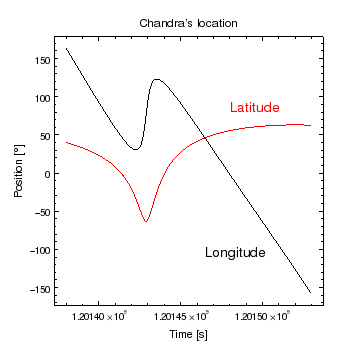### Figure 8: Moving the labels

The label positions have been changed to better match the curves they represent. The postscript output created by print_window has been expanded so that it fits a US letter page size (whilst keeping the aspect ratio fixed); if the fittopage option had not been set in the call then the page size would have matched the screen size (compare the postscript output from this figure with Figure 3).

The X axis tickformat has been changed to display a fixed number of decimal places - in this case 5 - by using the value "%.5z". The results can be compared to those in Figure 3, which used the tickformat of "%z".

The fourth argument to move_label is set to 1 to indicate that these are relative shifts rather than absolute positions (which is the default when the argument is not given or set to 0). The undo command is particularly useful here when trying to move items around!

Objects can also be selected in the ChIPS window by double clicking, which will surround the object with a set of handles. The object can then be dragged around with the mouse or by using the arrow keys, re-sized, or rotated, depending on the type of the object.

## Changing and fixing axis limits

We are now going to show how axis limits can be changed, and how this stops them from being automatically re-sized when new curves are added to the plot. To start with we use clear to remove all ChIPS windows and to re-set the numbering of objects (the erase command could also have been used to delete the contents of the window but leave the window itself). The set_preference call is used to change the default symbol style value for curves to "none" (this means that any new curves drawn in this session will only be drawn using lines connecting the points).

```chips> clear()
chips> set_preference("curve.symbol.style", "none")
chips> limits(Y_AXIS, -90, 90)
chips> print (info())
Window [win1]
Frame [frm1]
Plot [plot1]   (0.15,0.15)  .. (0.90,0.90)
Border bottom [bx1]  top [bx2]  left [by1]  right [by2]
Curve [crv1]
X Axis [ax1]
Y Axis [ay1]

```

### Figure 9: Changing the y-axis limits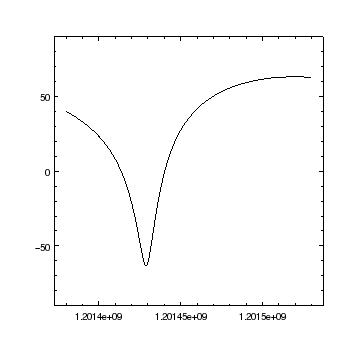### Figure 9: Changing the y-axis limits

The plot was created using add_curve, rather than make_figure, which means that no axis labels or plot title have been created. The y-axis limits have been changed by using the limits command. The symbol style for the curve does not have to be changed to "none", as it was in previous examples such as Figure 2 since the curve.symbol.style preference was changed to "none" before creating the curve.

The call to limits stops the Y-axis from re-sizing when a new curve is added, as shown below:

```chips> add_curve("pos.dat[cols utime,longitude]", ["line.color", "orange"])
chips> save_state("plot3.state")
```

### Figure 10: Adding a second curve when the y-axis limits are fixed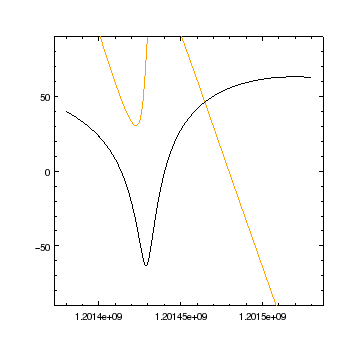### Figure 10: Adding a second curve when the y-axis limits are fixed

Although the second curve has a y range much larger than -90 to +90 (e.g. Figure 6), the y axis has not changed from the range used in Figure 9 since the values were fixed by the call to limits.

The auto-scaling can be returned by using the AUTO value for the minimum or maximum values: the first call below changes the axis so that it begins at -90 and ends at the maximum value of both curves (so only the maximum value is autoscaled), whereas the second call uses both the minimum and maximum values.

```chips> limits(Y_AXIS, -90, AUTO)
chips> limits(Y_AXIS, AUTO, AUTO)
```

At this point the y-axis range matches that of Figure 8, and is set to ymin - ydelta to ymax + ydelta, where ymin and ymax are the minimum and maximum y values of the two curves, ydelta = pad * (ymax - ymin) and pad is the fractional padding factor for the axis. The default value for pad is 0.05, so that there is a small gap between the end of the curve and the axes. This can be changed just like the curve color was changed:

```chips> print(get_plot_yrange())
[-173.835, 178.935]
chips> print(get_curve_yrange("crv2"))
[-157.8, 162.9]
0.05
chips> print(get_plot_yrange())
[-157.8, 162.9]
```

### Figure 11: Removing the padding on the y axis### Figure 11: Removing the padding on the y axis

By setting the pad attribute of the y axis to 0 the y axis now covers the same range as the curves (in this case the longitude values since they cover a larger range than the latitude curve).

The get_plot_yrange and get_curve_yrange routines return the data range covered by the plot axis and curve respectively. There are versions of these calls for the X axis and other objects.

The interactive capabilities of ChIPS allow you to change the plot limits from within the ChIPS GUI or directly from the window by dragging around the plot contents or zooming into or out of the plot.

## A more-complicated example

In this section we shall use Crates to read in data from a file, manipulate it, and then plot the new data using ChIPS. The aim is to plot the longitude, latitude, and altitude columns from pos.dat against time; for this we shall use two plots.

First we use the read_file and copy_colvals routines from Crates to read in the data. The return value from copy_colvals is a NumPy array. We define the x array to be the time, in kiloseconds, since the first entry, and then set up the lng, lat, and alt arrays with the data from the file.

```chips> cr = read_file("pos.dat")
chips> ut = copy_colvals(cr, "utime")
chips> print(ut)
[  1.20138018e+09   1.20138042e+09   1.20138060e+09 ...,   1.20152964e+09
1.20152976e+09   1.20152988e+09]
chips> x = (ut - ut)/1e3
chips> lng = copy_colvals(cr, "longitude")
chips> lat = copy_colvals(cr, "latitude")
chips> alt = copy_colvals(cr, "altitude")
```

### Create the first plot (longitude and latitude)

The first plot contains the longitude and latitude curves, and so is similar in construction to the plots above. The differences are that we explicitly create the plots, using the strip_chart command, and we use arrays, rather than a file name, with the add_curve calls.

```chips> clear()
chips> strip_chart(2)
chips> add_curve(x, lng, ["id", "lng", "line.color", "steelblue"])
chips> print (info())
Window [win1]
Frame [frm1]
Plot [plot1]   (0.15,0.52)  .. (0.90,0.90)
Border bottom [bx1]  top [bx2]  left [by1]  right [by2]
X Axis [ax1]
Y Axis [ay1]
Curve [lat]
Curve [lng]
Plot [plot2]   (0.15,0.15)  .. (0.90,0.52)
Border bottom [bx1]  top [bx2]  left [by1]  right [by2]
X Axis [ax1]
Y Axis [ay1]

```

### Figure 12: First plot (latitude and longitude)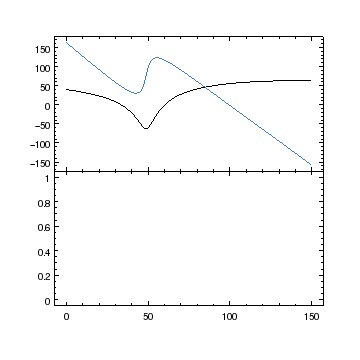### Figure 12: First plot (latitude and longitude)

The strip_chart call has created two plots arranged vertically, and set up so that the X axes of the two plots are linked together, so that changes to one are automatically reflected in the other plot (which is why the X axis on the bottom plot goes from 0 to 150 to match the data in the top plot).

The result of setting the "id" attribute in the add_curve call can be seen in the output of the info command; instead of crv1 and crv2 the curves have been labeled with meaningful identifiers. They can be used in set_curve calls as shown below:

```chips> set_curve("lat", ["symbol.style", "circle"])
chips> undo()
```

### Adding labels to the top plot

The next step is to add labels to identify the two lines. This time we use a different approach to that used in Figure 8, and place the labels in the bottom-left and top-right corners of the plot. To do this we place the labels using the "plot normalized" coordinate system, which ranges from (0,0) at the bottom-left of the plot area to (1,1) at the top-right of the plot. So, the "Latitude" label starts 5% into the plot area along the X axis and 10% along the Y axis, whereas the "Latitude" label is placed so that it ends at a position 95% along the X axis and 90% along the Y axis (the halign and valign attributes control the position of the reference position of the label along the string and the default values of 0 indicate the start of the string; for the latitude label we change this position so that it marks the end of the string). Since the labels are positioned with respect to the plot, and not the data values, they do not move when the Y axis range is changed by the limits call.

```chips> add_label(0.05, 0.1, "Latitude", ["id", "lat", "coordsys", PLOT_NORM])

chips> id = ChipsId()
chips> id.coord_sys = PLOT_NORM
chips> li = ChipsLabel()
chips> print (li)
angle = None
color = None
depth = None
font = None
fontstyle = None
halign = None
id = None
size = None
stem = None
valign = None

chips> li.id = "lng"
chips> li.color = "steelblue"
chips> li.halign = 1
chips> li.valign = 1
chips> add_label(id, 0.95, 0.9, "Longitude", li)
chips> limits(Y_AXIS, -180, 180)
```

The first label - called "lat" - is set use to plot-normalized coordinates using the coordsys attribute.

This example has used another way of controlling the appearance of an object, in this case for the second label - called "lng"; it is closely related to the get_<object> call, since the Chips<Object> call returns the same object but this time with all the fields empty, which means it can be used when you do not have an existing object (in this case label) in the plot. We could have used the following, without the line break, but it does not fit on a single line when this document is printed out:

add_label(0.95, 0.9, "Longitude", ["id", "lng", "coordsys", PLOT_NORM, "color", "steelblue", "halign", 1, "valign", 1])

### Figure 13: Adding labels in plot coordinates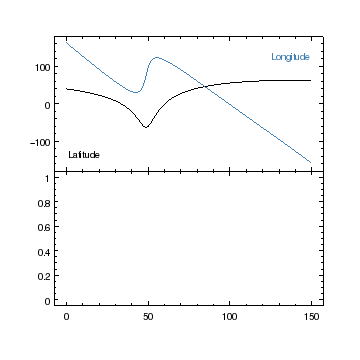### Figure 13: Adding labels in plot coordinates

The labels have been added using a plot-normalized coordinate system rather than the default data coordinate system (as used in Figure 8, for example). They could have been placed using data coordinates in this figure but it is easier to get the relative spacing correct in this example using plot coordinates (i.e. ensure that the gap from the start or end of the label is only 5% of the X axis and 10% of the Y axis).

The id attribute for each label was explicitly given, as it was with the curves, rather than use the default value. Note that the same labels can be used for different object types (here curves and labels). The output of the info command at this point is:

```chips> print(info())
Window [win1]
Frame [frm1]
Plot [plot1]   (0.15,0.52)  .. (0.90,0.90)
Border bottom [bx1]  top [bx2]  left [by1]  right [by2]
X Axis [ax1]
Y Axis [ay1]
Curve [lat]
Curve [lng]
Label [lat]
Label [lng]
Plot [plot2]   (0.15,0.15)  .. (0.90,0.52)
Border bottom [bx1]  top [bx2]  left [by1]  right [by2]
X Axis [ax1]
Y Axis [ay1]

```

### Finishing off the first plot

We decide to change the spacing of the major and minor tick marks of the Y axis - so that a major tick mark appears every 90 degrees and that there are two minor tick marks between every major one - and add a label to the Y axis.

```chips> set_yaxis(["majortick.interval", 90, "minortick.count", 2])
chips> set_plot_ylabel ("Degrees")
```

### Figure 14: Adjusting the y-axis labels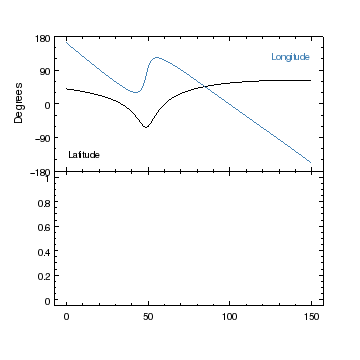### Figure 14: Adjusting the y-axis labels

The spacing of the major and minor tick marks along the Y axis have been changed to 90 and 30 degrees respectively.

### Adding a curve to the second plot

The info output shows that the second plot is called plot2, so we can use the current_plot command to change to this plot. This means that any new objects that are created (and that belong to a plot, such as a curve) will appear in the second plot rather than the first one.

```chips> print(info_current())
Window [win1]
Frame [frm1]
Plot [plot1]
X Axis [ax1]
Y Axis [ay1]
Curve [lng]
Label [lng]
Coord Sys [Data]
Coord Sys ID [ds2.2.2.12]

chips> current_plot("plot2")
chips> print (info_current())
Window [win1]
Frame [frm1]
Plot [plot2]
X Axis [ax1]
Y Axis [ay1]
Coord Sys [Data]
Coord Sys ID [ds2.2.3.13]

chips> log_scale(Y_AXIS)
chips> limits(Y_AXIS, 10000, 200000)
```

### Figure 15: Adding a curve to the second plot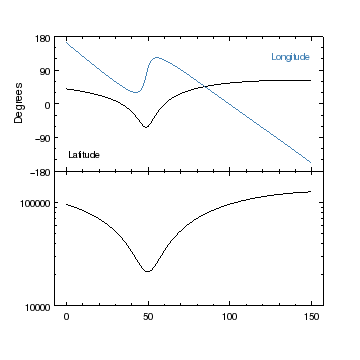### Figure 15: Adding a curve to the second plot

The altitude versus time data has been added to the second plot, and the Y axis changed to a logarithmic scale using the log_scale command. Note that the limits can be changed after the axis scaling has been changed.

The output of the info_current command was included above to show how it changes when you change to a different object: in this case the "lng" curve and "lng" label were no longer current since they belong to the first plot ("plot1").

### Changing the plot sizes

Now that we have added all the curves to the plots we make several final additions and adjustments before printing it out:

• Axis labels are added. Since the X-axis label includes a LaTeX command to indicate the Delta symbol, the string is written as r"\Delta Time (ks)" to avoid Python from incorrectly interpreting the \D as a control character. An alternative would have been to say "\\Delta Time (ks)".

• A label is added to the top-left corner of the plot using the PLOT_NORM coordinate system.

• The adjust_grid_yrelsize command is used to make the plots in the first row twice the size of any other rows (in this case there are only two rows).

```chips> set_plot_xlabel(r"\Delta Time (ks)")
chips> set_plot_ylabel("km")
chips> add_label(0.05, 0.9, "Altitude", ["id", "alt",
"coordsys", PLOT_NORM, "valign", 1])
chips> print_window ("plot4", ["fittopage", True])
chips> print_window ("plot4.pdf", ["fittopage", True])
chips> print_window ("plot4.png")
chips> save_state("plot4.state")
```

### Figure 16: The final plot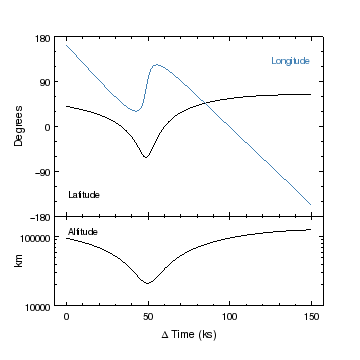### Figure 16: The final plot

The final version shows the longitude and latitude values in the top plot with the altitude values in the bottom plot, where all values are drawn as a function of time.

## How can I ...?

The other ChIPS threads provide further examples of how to use ChIPS, as do the examples in the gallery and the ChIPS FAQ.

### How can I use the GUI?

Please see either the GUI section of the "Starting ChIPS" thread or ahelp chipsgui for information on using the new GUI.

### How can I overwrite files?

ChIPS has a clobber preference setting which defaults to false; this means that ChIPS will not over-write a file, for example when calling print_window. This preference can be over-ridden temporarily, for instance:

```chips> print_window("plot")
chips ERROR: The output file /data/work/plot.ps already exists but export.clobber is set to false
chips> print_window("plot", ["clobber", True])
```

or, by using set_preference, it can be changed for the whole session:

```chips> set_preference("export.clobber", "true")
```

Note that the save_state command currently does not accept options, so you can not say save_state(file, ["clobber", True]) and will have to either manually delete any existing files or use the set_preference call to change the clobber setting.

### Can I save this plot to work on at a later time?

ChIPS allows you to save the current state to a file, so that it can be read in at a later time, even on a different computer. The command save_state will create a ChIPS state file, and has been used with several of the plots in this thread, for example when creating Figure 16:

```chips> save_state("plot4.state")
```

creates the file plot4.state which can be loaded into a ChIPS session (the same one or a different one, even on a completely different machine) by saying

```chips> load_state("plot4.state")
```

The state file support is different in CIAO 4 to that in CIAO 3.4, in particular:

• The file contains all the data that has been plotted, so it does not need access to the original data files. This means you can send a colleague just the state file and they can also work on the plot, or you can copy the state file between desktop and laptop computers without having to also take along the data files.

• The state file records the ChIPS commands used, so you can use undo and redo after loading in the file to move through the command history (although the history buffer is not infinite, so not all commands will be able to be undone).

• The state file is stored in a binary format and so should not be opened in a text editor.

The make_script command creates a Python script that will re-create the current visualization. For example:

```chips> make_script("myplots.py")
```

will create the file myplots.py. This can be used in the same, or different, ChIPS session to re-create the visualization by using it to start ChIPS: e.g.

```unix% chips myplots.py
```

or from within ChIPS:

```chips> execfile("myplots.py")
```

The differences to the file created by save_state() are:

• The file can be edited by users since it is a normal Python, ASCII, file.

• The file does not record all the commands used to create the plots, instead it re-creates the visualization using ChIPS commands.

The File/Save As menu of the ChIPS GUI allows you to save either type of file (i.e. Python or binary state).

## Summary

• The make_figure command can be used to plot data from a file. The add_curve command can plot data from a file -

```chips> add_curve("pos.dat[altitude>100000][cols utime,latitude]")
```

- or Python array data:

```chips> x = np.arange(1,10)
```

When using a file name, the Data Model filtering syntax can be used, and files can be either FITS or ASCII format.

• The make_figure command will create a new plot whenever it is called. This means that it can not be used to add data to an existing plot. The add_curve command will create a plot, but only if there is no current plot, which means that it can be used to add more data to a plot.

• The undo and redo commands are very useful when a command doesn't do what you expected it too (or you have accidentally deleted the window).

• The GUI provides an easy way to modify your ChIPS visualizations, and the ability to add annotations such as labels and lines.

• The get_preference command returns a string which lists the default attributes of an object; these are the values that are used the next time that an object of this class is created. The set_preference command is used to change the values in this list.

• When creating an object - e.g. with add_curve or add_label - you can override the preference settings for that object. This can be done in one of four ways:

1. As a list of "attribute,value" pairs: for example

`chips> add_label(x, y, l, ["color", "red", "angle", 45])`
2. As a dictionary of "atribute,value" pairs: for example

`chips> add_label(x, y, l, {"color": "red", "angle": 45})`

This format was not used in this thread.

3. As a single string (with space-separated sets of "attribute=value"): for example

`add_label(x, y, l, "color=red angle=45")`

This format was not used in this thread.

4. The Chips<Object> call returns a variable which contains all the atributes of the object. After changing the attributes, the variable can be used in the add_<object> call.

```chips> ci = ChipsLabel()
chips> ci.color = "red"
chips> ci.angle = 45
```
• Labels, lines, regions, and points can be positioned using a range of coordinate systems:

Label Description
DATA The coordinate system defined by the data.
PLOT_NORM plot normalized, where (0,0) is the bottom-left of the plot and (1,1) is the top-right of the plot
FRAME_NORM frame normalized, where (0,0) is the bottom-left of the frame and (1,1) is the top-right of the frame

The coordinate system can be specified using the coord_sys field of the ChipsId object, or by setting the coordsys attribute in the add_<object> call.

• The get_<object> call returns a variable which contains the attribute settings for the current object:

```chips> print(get_label())
angle = 45.0
color = red
depth = 100
font = helvetica
fontstyle = normal
halign = 0.0
id = None
size = 12
stem = None
valign = 0.0
```
• There are six ways to change the attributes of an existing object (although the last two are very similar to each other):

1. With the ChIPS GUI (e.g. Figure 4).

2. The values can be given as a list of "attribute, value" pairs in a call to set_<object>:

```chips> set_curve(["line.color", "red", "symbol.style", "none"])
```
3. The values can be given as a dictionary of "attribute, value" pairs in a call to set_<object>:

```chips> set_curve({"line.color": "red", "symbol.style": "none"})
```

This format was not used in this thread.

4. The values can be given as a single string of space-separated "attribute=value" pairs:

```chips> set_curve("line.color=red symbol.style=none")
```

This format was not used in this thread.

5. The return value from get_<object> can be changed and then sent to set_<object>.

```chips> ci = get_curve()
chips> ci.line.color = "red"
chips> ci.symbol.style = "none"
chips> set_curve(ci)
```
6. The return value from Chips<Object> can be changed and then sent to set_<object>.

```chips> ci = ChipsCurve()
chips> ci.line.color = "red"
chips> ci.symbol.style = "none"
chips> set_curve(ci)
```
• ### Figure 17: Line Styles### Figure 17: Line Styles

There are a range of line styles that can be used with curves. The symbol support for curves thread shows other ways that curves can be drawn.

These lines can also be drawn at different thicknesses, as shown in Figure 5

• The info command returns a string which lists what objects have been created and how they are related. The info_current command is useful when multiple objects - such as curves in a plot or plots in a window - have been created, since it lists the objects that ChIPS will act on when calls like set_<object> are made without specifying an identifier.

All objects have a name (the value within the "[]" in the info output); if not specified when the object is created (by use of the id attribute) then the name will default to the stem preference setting for that object followed by a number; examples include crv1 and plot2.

• The print_window command is used to create a "hardcopy" version of the window. The default output format is postscript but encapsulated postscript, PDF, PNG, and JPEG formats are also supported.

There is a GUI for printing, which can be created from the command line, by setting the printdialog attribite, or directly from the ChIPS GUI.

The postscript and PDF output formats can be re-sized using the fittopage, pagesize, and keepaspect attributes, so

`print_window("plot", ["fittopage", True])`

will create plot.ps where the contents have been expanded to fill a US letter size page whilst retaining its aspect ratio.

When using the standard preference settings, the on-screen display will use white lines, text, and symbols on a black background which are automatically reversed by the print_window call, so that the PS, EPS, PDF, PNG, and JPEG formats are drawn in black on a white background. This happens for any color value that is set to default; this means that

`set_curve(["line.color", "white"])`

will use a white line for the curve on the screen and when print_window is called.

The print format is taken from the suffix of the file name, if given. So

```chips> print_window("plot.pdf")
chips> print_window("plot.png")
```

create PDF and PNG formats of the visualization respectively. The format attribute is used if there is no suffix, or it is not recognized.

## History

 09 Jul 2008 New for CIAO 4.0 15 Dec 2008 CIAO 4.1 - use copy_colvals rather than get_colvals; output of info has changed for labels; labels can now be positioned using the coordsys attribute; noted that state files from CIAO 4.0 are not readable by CIAO 4.1. 15 Dec 2009 Updated for CIAO 4.2: the output format for print_window is now taken from the suffix of the filename (Figure 3), curves have a new preference/attribute setting (err.caplength), and the new command make_script can be used to create a Python script that re-creates the current visualization. 15 Dec 2010 Updated for CIAO 4.3: the info output has changed slightly; the order of objects within a plot may have changed and the auto-generated names of coordinate systems has cahnged from strings like "plot1_ax1ay2" to ones like "ds2.2.3.13". 15 Dec 2011 Updated for CIAO 4.4: highlighted the new ChIPS GUI in multiple places (e.g. Figure 4). 13 Dec 2012 Updated for CIAO 4.5. 04 Dec 2013 Updated for CIAO 4.6. 10 Dec 2014 Updated for CIAO 4.7. 15 Dec 2015 Updated for CIAO 4.8.